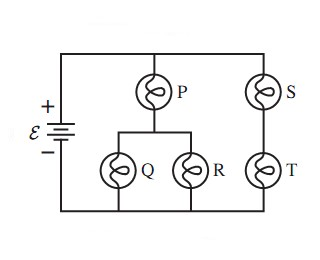# Problem: The five identical bulbs in the following figure are all glowing. The battery is ideal.What is the order of brightness of the bulbs, from brightest to dimmest? Some may be equal.A. P = S = T &gt; Q = RB. P &gt; S = T &gt; Q = RC. P &gt; Q = R &gt; S = TD. P = S &gt; Q = R = T

###### FREE Expert Solution

The brightness of a bulb is determined by power.

Power:

$\overline{){\mathbf{P}}{\mathbf{=}}{{\mathbf{i}}}^{{\mathbf{2}}}{\mathbf{R}}}$

Since the bulbs are identical, the resistance in each is the same.

Therefore, the power depends on the current passing through each bulb only.

87% (383 ratings)###### Problem Details

The five identical bulbs in the following figure are all glowing. The battery is ideal.What is the order of brightness of the bulbs, from brightest to dimmest? Some may be equal.

A. P = S = T > Q = R

B. P > S = T > Q = R

C. P > Q = R > S = T

D. P = S > Q = R = T